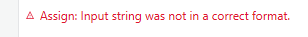# Select query in a data table where column name is integer

I have a column with the name 23 in my data table. I’m not able to run select query with that column name as shown in above screenshot. If the correct syntax is provided, the above query should return 1 as result. But it’s not working with my query. How to solve this?

Hi,

Can you try the following LINQ expression instead of Select method?

``````MainExcelDT.AsEnumerable.Count(Function(r) r("Name of mentor").ToString="Dr. S. VENKATESWARLU" AndAlso r("COLLEGE").ToString="GPCET" AndAlso r("BRANCH").ToString="MEC" AndAlso Int32.Parse(r("Year").ToString)=3 AndAlso Int32.Parse(r("23").ToString)>65)
``````

Regards,

1 Like

ok. I’ll try that. Can I also use variables instead of values in this query?

Hi,

Yes, as the following.

``````MainExcelDT.AsEnumerable.Count(Function(r) r("Name of mentor").ToString=strDrName AndAlso r("COLLEGE").ToString=strCollegeName AndAlso r("BRANCH").ToString=strBranchName AndAlso Int32.Parse(r("Year").ToString)=intYear AndAlso Int32.Parse(r("23").ToString)>int23Value)
``````

Regards,

1 Like

Hi,

In my environment, I got same error in immediate panel. However it works in activity such as MesageBox, for example. Can you try the expression in an activity?

Regards,

Just pass your result into a List(convert to a list) then check the count. List work with if there no matches but DT are don’t work that way

Ok. I’ll try

Did you mean that I should convert entire data table to list

In your LINQ query convert result to list by using .ToList function and after that check the result count. basically goal is convert your LINQ result into a list and after that check the count

1 Like

When I tried in Assign activity, I’m getting error:as per this image

sorry do not run the Count function just run SELECT or WHERE function and pass it as list and take the count

Hi,

It seems there are non-int32 value (include blank) in Year and/or 23 column.
So, for now, can you try the following expression?

``````MainExcelDT.AsEnumerable.Count(Function(r) r("Name of mentor").ToString=strDrName AndAlso r("COLLEGE").ToString=strCollegeName AndAlso r("BRANCH").ToString=strBranchName AndAlso  Int32.TryParse(r("Year").ToString,New Int32) AndAlso Int32.Parse(r("Year").ToString)=intYear AndAlso Int32.TryParse(r("23").ToString,New Int32) AndAlso Int32.Parse(r("23").ToString)>int23Value)
``````

Regards,

1 Like

I’m not sure if this works. I’ll convert my column name to string like day 23. Then it will work

I converted my column name to Day 23 and tried the following. Nothing works